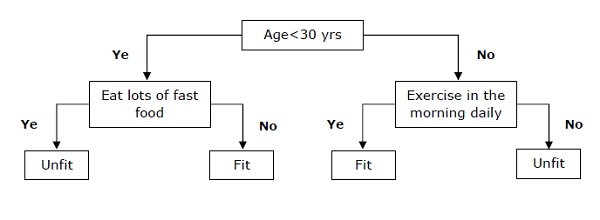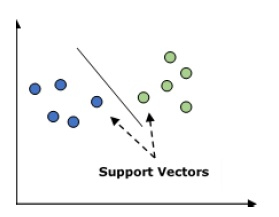# Machine Learning Algorithm Posted on: 18/10/19 Category: Data Science, Technology Update

Basically, the main focus of machine learning is to allow the computers learn automatically without human intervention. Now the question arises that how such learning can be started and done? It can be started with the observations of data. The data can be some examples, instruction or some direct experiences too. Then on the basis of this input, machine makes better decision by looking for some patterns in data.

## Types of Machine Learning (ML)

Machine Learning Algorithms helps computer system learn without being explicitly programmed. These algorithms are categorized into supervised or unsupervised. Let us now see a few algorithms −

### Supervised machine learning algorithms

This is the most commonly used machine learning algorithm. It is called supervised because the process of algorithm learning from the training dataset can be thought of as a teacher supervising the learning process. In this kind of ML algorithm, the possible outcomes are already known and training data is also labeled with correct answers. It can be understood as follows −

Suppose we have input variables x and an output variable y and we applied an algorithm to learn the mapping function from the input to output such as −

Y = f(x)


Now, the main goal is to approximate the mapping function so well that when we have new input data (x), we can predict the output variable (Y) for that data.

Mainly supervised leaning problems can be divided into the following two kinds of problems −

• Classification − A problem is called classification problem when we have the categorized output such as “black”, “teaching”, “non-teaching”, etc.
• Regression − A problem is called regression problem when we have the real value output such as “distance”, “kilogram”, etc.

Decision tree, random forest, knn, logistic regression are the examples of supervised machine learning algorithms.

### Unsupervised machine learning algorithms

As the name suggests, these kinds of machine learning algorithms do not have any supervisor to provide any sort of guidance. That is why unsupervised machine learning algorithms are closely aligned with what some call true artificial intelligence. It can be understood as follows −

Suppose we have input variable x, then there will be no corresponding output variables as there is in supervised learning algorithms.

In simple words, we can say that in unsupervised learning there will be no correct answer and no teacher for the guidance. Algorithms help to discover interesting patterns in data.

Unsupervised learning problems can be divided into the following two kinds of problem −

• Clustering − In clustering problems, we need to discover the inherent groupings in the data. For example, grouping customers by their purchasing behavior.
• Association − A problem is called association problem because such kinds of problem require discovering the rules that describe large portions of our data. For example, finding the customers who buy both x and y.

K-means for clustering, Apriori algorithm for association are the examples of unsupervised machine learning algorithms.

### Reinforcement machine learning algorithms

These kinds of machine learning algorithms are used very less. These algorithms train the systems to make specific decisions. Basically, the machine is exposed to an environment where it trains itself continually using the trial and error method. These algorithms learn from past experience and tries to capture the best possible knowledge to make accurate decisions. Markov Decision Process is an example of reinforcement machine learning algorithms.

## Most Common Machine Learning Algorithms

In this section, we will learn about the most common machine learning algorithms. The algorithms are described below −

### Linear Regression

It is one of the most well-known algorithms in statistics and machine learning.

Basic concept − Mainly linear regression is a linear model that assumes a linear relationship between the input variables say x and the single output variable say y. In other words, we can say that y can be calculated from a linear combination of the input variables x. The relationship between variables can be established by fitting a best line.

### Types of Linear Regression

Linear regression is of the following two types −

• Simple linear regression − A linear regression algorithm is called simple linear regression if it is having only one independent variable.
• Multiple linear regression − A linear regression algorithm is called multiple linear regression if it is having more than one independent variable.

Linear regression is mainly used to estimate the real values based on continuous variable(s). For example, the total sale of a shop in a day, based on real values, can be estimated by linear regression.

### Logistic Regression

It is a classification algorithm and also known as logit regression.

Mainly logistic regression is a classification algorithm that is used to estimate the discrete values like 0 or 1, true or false, yes or no based on a given set of independent variable. Basically, it predicts the probability hence its output lies in between 0 and 1.

### Decision Tree

Decision tree is a supervised learning algorithm that is mostly used for classification problems.

Basically it is a classifier expressed as recursive partition based on the independent variables. Decision tree has nodes which form the rooted tree. Rooted tree is a directed tree with a node called “root”. Root does not have any incoming edges and all the other nodes have one incoming edge. These nodes are called leaves or decision nodes. For example, consider the following decision tree to see whether a person is fit or not.### Support Vector Machine (SVM)

It is used for both classification and regression problems. But mainly it is used for classification problems. The main concept of SVM is to plot each data item as a point in n-dimensional space with the value of each feature being the value of a particular coordinate. Here n would be the features we would have. Following is a simple graphical representation to understand the concept of SVM −In the above diagram, we have two features hence we first need to plot these two variables in two dimensional space where each point has two co-ordinates, called support vectors. The line splits the data into two different classified groups. This line would be the classifier.

### Naïve Bayes

It is also a classification technique. The logic behind this classification technique is to use Bayes theorem for building classifiers. The assumption is that the predictors are independent. In simple words, it assumes that the presence of a particular feature in a class is unrelated to the presence of any other feature. Below is the equation for Bayes theorem −

$$P\left ( \frac{A}{B} \right ) = \frac{P\left ( \frac{B}{A} \right )P\left ( A \right )}{P\left ( B \right )}$$

The Naïve Bayes model is easy to build and particularly useful for large data sets.

### K-Nearest Neighbors (KNN)

It is used for both classification and regression of the problems. It is widely used to solve classification problems. The main concept of this algorithm is that it used to store all the available cases and classifies new cases by majority votes of its k neighbors. The case being then assigned to the class which is the most common amongst its K-nearest neighbors, measured by a distance function. The distance function can be Euclidean, Minkowski and Hamming distance. Consider the following to use KNN −

• Computationally KNN are expensive than other algorithms used for classification problems.
• The normalization of variables needed otherwise higher range variables can bias it.
• In KNN, we need to work on pre-processing stage like noise removal.

### K-Means Clustering

As the name suggests, it is used to solve the clustering problems. It is basically a type of unsupervised learning. The main logic of K-Means clustering algorithm is to classify the data set through a number of clusters. Follow these steps to form clusters by K-means −

• K-means picks k number of points for each cluster known as centroids.
• Now each data point forms a cluster with the closest centroids, i.e., k clusters.
• Now, it will find the centroids of each cluster based on the existing cluster members.
• We need to repeat these steps until convergence occurs.

### Random Forest

It is a supervised classification algorithm. The advantage of random forest algorithm is that it can be used for both classification and regression kind of problems. Basically it is the collection of decision trees (i.e., forest) or you can say ensemble of the decision trees. The basic concept of random forest is that each tree gives a classification and the forest chooses the best classifications from them. Followings are the advantages of Random Forest algorithm −

• Random forest classifier can be used for both classification and regression tasks.
• They can handle the missing values.
• It won’t over fit the model even if we have more number of trees in the forest.